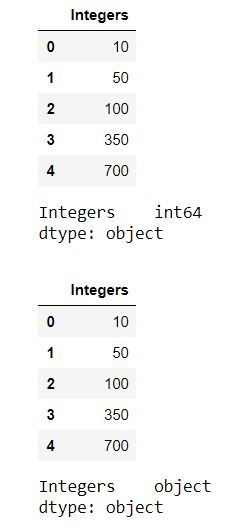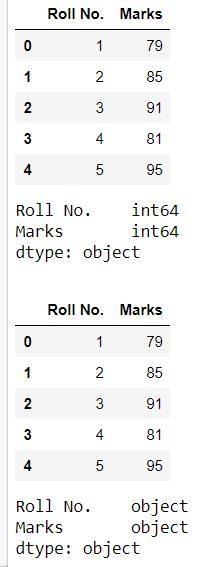# How to Convert Integers to Strings in Pandas DataFrame?

• Last Updated : 01 Aug, 2020

In this article, we’ll look at different methods to convert an integer into a string in a Pandas dataframe. In Pandas, there are different functions that we can use to achieve this task :

• map(str)
• astype(str)
• apply(str)
• applymap(str)

Example 1 : In this example, we’ll convert each value of a column of integers to string using the `map(str)` function.

 `# importing pandas as pd``import` `pandas as pd `` ` `# creating a dictionary of integers``dict` `=` `{``'Integers'` `: [``10``, ``50``, ``100``, ``350``, ``700``]}`` ` `# creating dataframe from dictionary``df ``=` `pd.DataFrame.from_dict(``dict``)``print``(df)``print``(df.dtypes)`` ` `print``(``'\n'``)`` ` `# converting each value of column to a string``df[``'Integers'``] ``=` `df[``'Integers'``].``map``(``str``)``print``(df)``print``(df.dtypes)`

Output :We can see in the above output that before the the datatype was `int64 `and after the conversion to a string, the datatype is an `object `which represents a string.

Example 2 : In this example, we’ll convert each value of a column of integers to string using the `astype(str) `function.

 `# importing pandas as pd``import` `pandas as pd `` ` `# creating a dictionary of integers``dict` `=` `{``'Integers'` `: [``10``, ``50``, ``100``, ``350``, ``700``]}`` ` `# creating dataframe from dictionary``df ``=` `pd.DataFrame.from_dict(``dict``)``print``(df)``print``(df.dtypes)`` ` `print``(``'\n'``)`` ` `# converting each value of column to a string``df[``'Integers'``] ``=` `df[``'Integers'``].astype(``str``)`` ` `print``(df)``print``(df.dtypes)`

Output :We can see in the above output that before the the datatype was `int64 `and after the conversion to a string, the datatype is an `object `which represents a string.

Example 3 : In this example, we’ll convert each value of a column of integers to string using the `apply(str)` function.

 `# importing pandas as pd``import` `pandas as pd `` ` `# creating a dictionary of integers``dict` `=` `{``'Integers'` `: [``10``, ``50``, ``100``, ``350``, ``700``]}`` ` `# creating dataframe from dictionary``df ``=` `pd.DataFrame.from_dict(``dict``)``print``(df)``print``(df.dtypes)`` ` `print``(``'\n'``)`` ` `# converting each value of column to a string``df[``'Integers'``] ``=` `df[``'Integers'``].``apply``(``str``)``print``(df)``print``(df.dtypes)`

Output :We can see in the above output that before the the datatype was `int64 `and after the conversion to a string, the datatype is an `object `which represents a string.

Example 4 : All the methods we saw above, convert a single column from an integer to a string. But we can also convert the whole dataframe into a string using the `applymap(str)` method.

 `# importing pandas as pd``import` `pandas as pd `` ` `# creating a dictionary of integers``dict` `=` `{``'Roll No.'` `: [``1``, ``2``, ``3``, ``4``, ``5``], ``'Marks'``:[``79``, ``85``, ``91``, ``81``, ``95``]}`` ` `# creating dataframe from dictionary``df ``=` `pd.DataFrame.from_dict(``dict``)``print``(df)``print``(df.dtypes)`` ` `print``(``'\n'``)`` ` `# converting each value of column to a string``df ``=` `df.applymap(``str``)``print``(df)``print``(df.dtypes)`

Output :We can see in the above output that before the the datatype was `int64 `and after the conversion to a string, the datatype is an `object `which represents a string.

My Personal Notes arrow_drop_up